• weigh
千次阅读 热门讨论
2020-12-06 20:29:37

# 一、 摘要

（没有具体研究实例分割，所以本博客并没有过多关心关于分割的模型与任务）
有些深度网络需要多任务来同时进行多个回归和分类目标，经过观察发现给予不同任务一个损失权重极大的影响着总体任务的表现。但是人工的搜索这个权重是极其困难和消费资源的，为此本论文提出了一个考虑每个任务同方差的不确定性来学习这些权重，这允许我们在分类和回归设置中同时学习不同单位或尺度的不同参数。实验证明本模型可以学习多任务权重，并且优于进行单任务独立建模训练。

创新：

1. 利用同方差不确定性同时学习不同数量和单元的分类和回归损失的一种新颖多任务损失
2. 建立统一的组合语义分割、定位分割和深度回归体系结构
3. 证明模型损失权重的重要性，并且能够解决并获得良好的参数。

# 二、 介绍

多任务学习的目的是通过从一个共享表示中学习多个目标来提高学习效率和预测精度。

以前同时学习多个任务的方法使用一个朴素的加权损失总和，其中损失的权重是一致的，或者手动调整。但是我们发现这个模型的表现很大程度依赖于这些权重。人工微调以及搜索来优化网络十分昂贵且难以解决本质问题，每个任务的最佳权重取决于测量尺度(例如米、厘米或毫米)和最终任务噪声的大小。

作者将同方差不确定性解释为任务相关的加权，并展示如何推导一个有原则的多任务损失函数，该函数可以学会平衡各种回归和分类损失。

# 三、 多任务学习

以前主要任务的简要表达式：
L total = ∑ i w i L i (1) L_{\text {total}}=\sum_{i} w_{i} L_{i} \tag{1}
只是简单的对每个子任务线性求和，但是这样存在很多问题，例如模型对于权重参数很敏感，如下图2: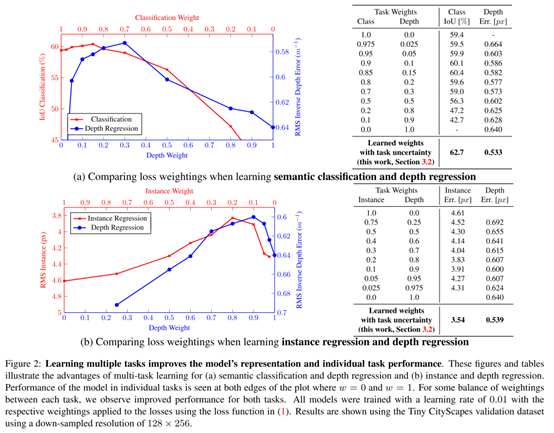## 1. 同方差不确定性

在贝叶斯模型中，不确定性主要有两种类型：

1. 认知不确定性是模型中的不确定性，它捕获了我们的模型由于缺乏训练数据而不知道的东西，这可以用增加的训练数据来解释。
2. 任意不确定性抓住了我们对数据无法解释的信息的不确定性。任意不确定性可以用观察所有解释变量的能力来解释，而且精确度越来越高。

任意不确定性又可分为两种：

1. 依赖数据或异方差不确定性，它依赖于输入数据，并被预测为模型输出。
2. 任务依赖或同方差不确定性，它不依赖于输入数据。它不是一个模型输出，而是一个对所有输入数据保持不变，并在不同任务之间变化的数量。因此，它可以被描述为任务依赖的不确定性

在多任务学习问题中，我们可以使用同方差不确定性作为加权损失的基础。

## 2. 多任务似然回归

在本节中，推导了一个基于同方差不确定性高斯似然最大化的多任务损失函数。让 f W ( x ) f^W (x) 表示输入 x x 在基于参数 W W 的神经网络的输出。定义以下概率模型，对于回归任务，将概率定义为高斯分布，其均值由模型输出给出：
p ( y ∣ f W ( x ) ) = N ( f W ( x ) , σ 2 ) (2) p\left(\mathbf{y} \mid \mathbf{f}^{\mathbf{W}}(\mathbf{x})\right)=\mathcal{N}\left(\mathbf{f}^{\mathbf{W}}(\mathbf{x}), \sigma^{2}\right)\tag{2}

噪声标量 σ σ ，代表输出需要携带多少噪声。

为了进行分类，我们经常通过 s o f t m a x softmax 函数压缩模型输出，由概率向量得到的样本：
p ( y ∣ f W ( x ) ) = Softmax ⁡ ( f W ( x ) ) (3) p\left(\mathbf{y} \mid \mathbf{f}^{\mathbf{W}}(\mathbf{x})\right)=\operatorname{Softmax}\left(\mathbf{f}^{\mathbf{W}}(\mathbf{x})\right) \tag{3}
在多个模型输出的情况下，通常定义对输出进行因式分解的可能性，给出一些充分的统计信息。我们定义 f W ( x ) f^W (x) 作为我们的充分统计量，并获得以下多任务似然：
p ( y 1 , … , y K ∣ f W ( x ) ) = p ( y 1 ∣ f W ( x ) ) … p ( y K ∣ f W ( x ) ) (4) p\left(\mathbf{y}_{1}, \ldots, \mathbf{y}_{K} \mid \mathbf{f}^{\mathbf{W}}(\mathbf{x})\right)=p\left(\mathbf{y}_{1} \mid \mathbf{f}^{\mathbf{W}}(\mathbf{x})\right) \ldots p\left(\mathbf{y}_{K} \mid \mathbf{f}^{\mathbf{W}}(\mathbf{x})\right) \tag{4}

y 1 , … , y K y_1,…,y_K 为模型（例如语义分割，深度回归）输出。

最大似然推导中，最大化模型的对数似然。

例如回归任务中，对数似然可以被表达为：
log ⁡ p ( y ∣ f W ( x ) ) ∝ − 1 2 σ 2 ∥ y − f W ( x ) ∥ 2 − log ⁡ σ (5) \log p\left(\mathbf{y} \mid \mathbf{f}^{\mathbf{W}}(\mathbf{x})\right) \propto-\frac{1}{2 \sigma^{2}}\left\|\mathbf{y}-\mathbf{f}^{\mathbf{W}}(\mathbf{x})\right\|^{2}-\log \sigma \tag{5}

然后，我们最大化和模型参数 W W 和噪声参数 σ σ 有关的对数似然

假设模型输出由两向量 y 1 y_1 y 2 y_2 组成（两个回归任务），服从高斯分布：
p ( y 1 , y 2 ∣ f W ( x ) ) = p ( y 1 ∣ f W ( x ) ) ⋅ p ( y 2 ∣ f W ( x ) ) = N ( y 1 ; f W ( x ) , σ 1 2 ) ⋅ N ( y 2 ; f W ( x ) , σ 2 2 ) (6) \begin{aligned} p\left(\mathbf{y}_{1}, \mathbf{y}_{2} \mid \mathbf{f}^{\mathbf{W}}(\mathbf{x})\right) &=p\left(\mathbf{y}_{1} \mid \mathbf{f}^{\mathbf{W}}(\mathbf{x})\right) \cdot p\left(\mathbf{y}_{2} \mid \mathbf{f}^{\mathbf{W}}(\mathbf{x})\right) \\ &=\mathcal{N}\left(\mathbf{y}_{1} ; \mathbf{f}^{\mathbf{W}}(\mathbf{x}), \sigma_{1}^{2}\right) \cdot \mathcal{N}\left(\mathbf{y}_{2} ; \mathbf{f}^{\mathbf{W}}(\mathbf{x}), \sigma_{2}^{2}\right) \end{aligned} \tag{6}

这导致了我们多任务模型的最小化目标 L ( W , σ 1 , σ 2 ) \mathcal{L}(W,σ_1,σ_2)
= − log ⁡ p ( y 1 , y 2 ∣ f W ( x ) ) ∝ 1 2 σ 1 2 ∥ y 1 − f W ( x ) ∥ 2 + 1 2 σ 2 2 ∥ y 2 − f W ( x ) ∥ 2 + log ⁡ σ 1 σ 2 = 1 2 σ 1 2 L 1 ( W ) + 1 2 σ 2 2 L 2 ( W ) + log ⁡ σ 1 σ 2 (7) \begin{aligned} &=-\log p\left(\mathbf{y}_{1}, \mathbf{y}_{2} \mid \mathbf{f}^{\mathbf{W}}(\mathbf{x})\right)\\ &\propto \frac{1}{2 \sigma_{1}^{2}}\left\|\mathbf{y}_{1}-\mathbf{f}^{\mathbf{W}}(\mathbf{x})\right\|^{2}+\frac{1}{2 \sigma_{2}^{2}}\left\|\mathbf{y}_{2}-\mathbf{f}^{\mathbf{W}}(\mathbf{x})\right\|^{2}+\log \sigma_{1} \sigma_{2}\\ &=\frac{1}{2 \sigma_{1}^{2}} \mathcal{L}_{1}(\mathbf{W})+\frac{1}{2 \sigma_{2}^{2}} \mathcal{L}_{2}(\mathbf{W})+\log \sigma_{1} \sigma_{2} \end{aligned} \tag{7}
L 1 ( W ) = ∥ y 1 − f w ( x ) ∥ 2 \mathcal{L}_{1}(\mathbf{W})=\left\|\mathbf{y}_{1}-\mathbf{f}^{\mathbf{w}}(\mathbf{x})\right\|^{2} 表示第一个输出变量 y 1 y_1 的损失， L 2 \mathcal{L}_{2} 同理。

参数 σ 1 , σ 2 σ_1,σ_2 相当于损失 L 1 ( W ) \mathcal L_1 (W) L 2 ( W ) \mathcal L_2 (W) 基于数据的自适应权重，因为当 σ 1 σ_1 （变量 y 1 y_1 的噪声）增加时， L 1 ( W ) \mathcal L_1 (W) 的权重下降。相应的噪声减小，对应损失权重上升。损失最后一项充当噪声的调节器，抑制噪声的过大增加。

这种构造可以简单地扩展到多元回归输出

对于分类函数，我们通过对缩放后的输出进行 s o f t m a x softmax 函数作为分类似然：
p ( y ∣ f W ( x ) , σ ) = Softmax ⁡ ( 1 σ 2 f W ( x ) ) (8) p\left(\mathbf{y} \mid \mathbf{f}^{\mathbf{W}}(\mathbf{x}), \sigma\right)=\operatorname{Softmax}\left(\frac{1}{\sigma^{2}} \mathbf{f}^{\mathbf{W}}(\mathbf{x})\right) \tag{8}
σ σ 是一个正的缩放值。可以理解为一个 B o l t z − m a n n Boltz- mann 分布（ G i b b s Gibbs 分布），输入由 σ 2 σ^2 进行缩放。这个缩放可以固定也可以被学习，其中参数的大小决定了离散分布的“均匀”(平坦)程度。这个输出的对数似然可以写成：
log ⁡ p ( y = c ∣ f W ( x ) , σ ) = 1 σ 2 f c W ( x ) − log ⁡ ∑ c ′ exp ⁡ ( 1 σ 2 f c ′ W ( x ) ) (9) \begin{aligned} \log p\left(\mathbf{y}=c \mid \mathbf{f}^{\mathbf{W}}(\mathbf{x}), \sigma\right) &=\frac{1}{\sigma^{2}} f_{c}^{\mathbf{W}}(\mathbf{x}) \\ &-\log \sum_{c^{\prime}} \exp \left(\frac{1}{\sigma^{2}} f_{c^{\prime}}^{\mathbf{W}}(\mathbf{x})\right) \end{aligned} \tag{9}
f c W ( x ) f_c^W (x) 表示向量 f W ( x ) f^W (x) 的第 c c 个元素。

假设模型多输出由一个连续输出 y 1 y_1 和离散输出 y 2 y_2 组成，分别由高斯似然和 s o f t m a x softmax 似然建模进行联合损失得 L ( W , σ 1 , σ 2 ) \mathcal L(W,σ_1, σ_2)
= − log ⁡ p ( y 1 , y 2 = c ∣ f W ( x ) ) = − log ⁡ N ( y 1 ; f W ( x ) , σ 1 2 ) ⋅ Softmax ⁡ ( y 2 = c ; f W ( x ) , σ 2 ) = 1 2 σ 1 2 ∥ y 1 − f W ( x ) ∥ 2 + log ⁡ σ 1 − log ⁡ p ( y 2 = c ∣ f W ( x ) , σ 2 ) = 1 2 σ 1 2 L 1 ( W ) + 1 σ 2 2 L 2 ( W ) + log ⁡ σ 1 + log ⁡ ∑ c ′ exp ⁡ ( 1 σ 2 2 f c ′ W ( x ) ) ( ∑ c ′ exp ⁡ ( f c ′ W ( x ) ) ) 1 σ 2 2 ≈ 1 2 σ 1 2 L 1 ( W ) + 1 σ 2 2 L 2 ( W ) + log ⁡ σ 1 + log ⁡ σ 2 (10) \begin{array}{l} =-\log p\left(\mathbf{y}_{1}, \mathbf{y}_{2}=c \mid \mathbf{f}^{\mathbf{W}}(\mathbf{x})\right) \\ =-\log \mathcal{N}\left(\mathbf{y}_{1} ; \mathbf{f}^{\mathbf{W}}(\mathbf{x}), \sigma_{1}^{2}\right) \cdot \operatorname{Softmax}\left(\mathbf{y}_{2}=c ; \mathbf{f}^{\mathbf{W}}(\mathbf{x}), \sigma_{2}\right) \\ =\frac{1}{2 \sigma_{1}^{2}}\left\|\mathbf{y}_{1}-\mathbf{f}^{\mathbf{W}}(\mathbf{x})\right\|^{2}+\log \sigma_{1}-\log p\left(\mathbf{y}_{2}=c \mid \mathbf{f}^{\mathbf{W}}(\mathbf{x}), \sigma_{2}\right) \\ =\frac{1}{2 \sigma_{1}^{2}} \mathcal{L}_{1}(\mathbf{W})+\frac{1}{\sigma_{2}^{2}} \mathcal{L}_{2}(\mathbf{W})+\log \sigma_{1} \\ \quad+\log \frac{\sum_{c^{\prime}} \exp \left(\frac{1}{\sigma_{2}^{2}} f_{c^{\prime}}^{\mathbf{W}}(\mathbf{x})\right)}{\left(\sum_{c^{\prime}} \exp \left(f_{c^{\prime}} \mathbf{W}(\mathbf{x})\right)\right)^{\frac{1}{\sigma_{2}^{2}}}} \\ \approx \frac{1}{2 \sigma_{1}^{2}} \mathcal{L}_{1}(\mathbf{W})+\frac{1}{\sigma_{2}^{2}} \mathcal{L}_{2}(\mathbf{W})+\log \sigma_{1}+\log \sigma_{2} \end{array} \tag{10}

L 1 ( W ) = ∥ y 1 − f w ( x ) ∥ 2 \mathcal{L}_{1}(\mathbf{W})=\left\|\mathbf{y}_{1}-\mathbf{f}^{\mathbf{w}}(\mathbf{x})\right\|^{2} 表示 y 1 y_1 的欧几里得损失， L 2 ( W ) = − log ⁡ S o f t m a x ( y 2 , f W ( x ) ) \mathcal L_2(W)=-\log Softmax(y_2,f^W(x)) 为关于 y 2 y_2 的交叉熵损失（ f W ( x ) f^W(x) 未被缩放）。使用 W W σ 1 σ_1 σ 1 σ_1 进行优化。最后的 ≈ ≈ ，当 σ 2 → 1 σ_2→1 时候趋近 = =

最后一个目标可以被看作是学习每个产出损失的相对权重。缩放值 σ 2 σ_2 越大，则损失 L 2 ( W ) \mathcal L_2 (W) 贡献越小，缩放受 log ⁡ σ 2 \log σ_2 规范，缩放太大，则目标会被惩罚。

如此，这个函数就可以被随意的由离散和连续损失函数进行组合，这个损失是平滑可微的，并且是很好的形式，使得任务权值不会收敛到零，不同于 ( 1 ) (1) 有可能将损失很快的收敛到0。

实验中，训练网络去预测 l o g log 变量 s ∶ = l o g σ 2 s{∶=} log σ_2 ，因为这个变量比直接回归 σ 2 σ_2 在数学上更加的稳定，损失避免了除以 0 0

# 四、 结论

证明了对损失项的正确加权对于多任务学习问题是至关重要的，同时证明了同方差(任务)不确定性是损失赋权的有效方法。算法 神经网络 深度学习 人工智能
更多相关内容
• =========================================================================================================================================================...-Weigh 10卡车票务软件的用户实时查看生产编号...
• application in BCX to find the odd ball from 12 identical balls in 3 weighes
• 1.背景 目前，很多应用中都因为用了多任务取得了不错的效果，那么如何将这些任务的loss有机组合在一起？ 一种简答粗暴方法就是手动调节多任务之间的loss的相对权重，然后加和，如下： 这种方式把权重作为超参调试的...

## 1.背景

目前，很多应用中都因为用了多任务取得了不错的效果，那么如何将这些任务的loss有机组合在一起？

一种简答粗暴方法就是手动调节多任务之间的loss的相对权重，然后加和，如下：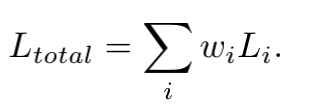这种方式把权重作为超参调试的方式，往往耗时耗力。

本文参考就如何动态设置多任务（分类与回归）之间的loss的相对权重做简单介绍。

## 2.解决方案

在贝叶斯建模中，认为模型有一些不确定性(随机变量)，可能是先验问题导致的，也有可能是后验问题导致的，大体可将其分为两种，如下：

• 认知不确定性：数据不足导致的模型学习不足的不确定性，比如：小学生去参加高考，很多知识都没学过，自然考不好。
• 偶然不确定性：噪声导致的不确定性

偶然不确定性又分为：

• 数据依赖不确定性（异方差）：输入数据的不确定性，导致输出不确定，比如：小明学习使用的教材部分有问题，导致小明考试考不到高分。
• 任务依赖不确定性（同方差）：不同任务自身的学习能力的不同，导致学习结果不确定性。比如：小明为了提高成绩，一方面努力学习知识概念，另一方面猛做练习题，他们都有各自的优势，但是也都有一定的局限性。其中，同方差 指的是假定数据输入一定的情况下，真实的分布与任务的输出之间有一个恒定的方差。

解决方案为什么考虑的用贝叶斯NN？贝叶斯建模中的不确定性能够表示不同任务之间的难易程度，能够很好的为任务的输出f，真实分布y以及方差δ之间建模。

动态loss权重就是考虑在同方差条件下，接下来我们如何建模展开。

## 3 建模

假设输入为 X X ，参数矩阵为 W W ，输出为 f W ( x ) f^W(x) ，真实分布为 y y

### 3.1 回归任务

对于回归任务，在给定其输出 f W ( x ) f^W(x) 的情况下 y y 的概率为高斯似然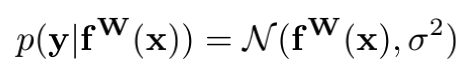(对于高斯似然， σ σ 为模型的观测噪声参数，表示输出数据中的噪声量)

f W ( x ) f^W(x) y y 代入上述高斯函数中，可得：
p ( y ∣ f W ( x ) ) = 1 2 π σ e − ( y − f W ( x ) ) 2 2 σ 2 p(y|f^W(x))=\frac{1}{\sqrt{2\pi}\sigma}e^{-\frac{(y-f^W(x))^2}{2\sigma^2}}

两边求对数，可得其对数似然函数，如下：

l o g p ( y ∣ f W ( x ) ) ∝ − ( y − f W ( x ) ) 2 2 σ 2 − l o g σ logp(y|f^W(x))\propto-\frac{(y-f^W(x))^2}{2\sigma^2}-log\sigma

### 3.2 分类任务

对于分类任务，为了建立起与 σ σ 的关系，其概率描述为softmax的一种更为普遍的表示形式Boltzmann分布，也叫吉布斯分布：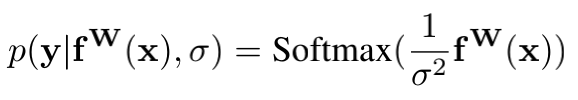系数 σ σ 可以是设定的，也可以是通过学习得到的，决定离散分布的平坦程度。该值和分布的不确定性(熵)有关。

同理，将 f W ( x ) f^W(x) y y 代入上述softmax函数中，可得对数似然函数，如下：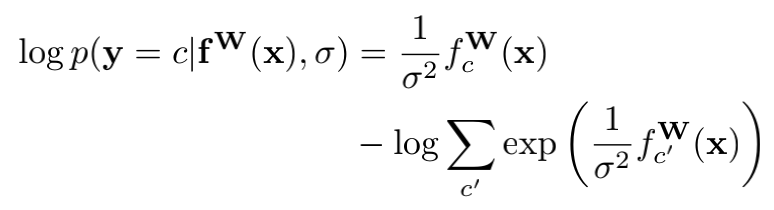其中，c为某一类别

### 3.3 多任务

对于回归与分类任务混合的多任务似然，假定 y 1 y_1 、…、 y k y_k 分别为回归任务和分类任务的真实输出，其似然为：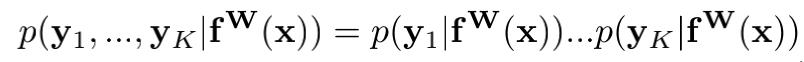那么，假设多任务的loss记作 L ( W , σ 1 , σ 2 ) L(W,\sigma_1 ,\sigma_2 ) ，那么则有：
= L ( W , σ 1 , σ 2 ) L(W,\sigma_1 ,\sigma_2 )
= − l o g N ( y 1 ; f W ( x ) , σ 1 2 ) ⋅ s o f t m a x ( y 2 = c ; f W ( x ) , σ 2 ) -logN(y_1;f^W(x),\sigma ^2_1)\cdot softmax(y_2=c;f^W(x),\sigma _2)
= 1 2 σ 1 2 ∥ y 1 − f W ( x ) ∥ 2 + l o g σ 1 − l o g p ( y 2 = c ∣ f W ( x ) , σ 2 ) \frac{1}{2\sigma ^2_1}\left \| y_1 - f^W(x) \right \|^2 + log\sigma_1 - logp(y_2=c|f^W(x),\sigma_2)
= 1 2 σ 1 2 ∥ y 1 − f W ( x ) ∥ 2 + l o g σ 1 − 1 σ 2 2 f c ′ W ( x ) + l o g ∑ c ′ e 1 σ 2 2 f c ′ W ( x ) \frac{1}{2\sigma ^2_1}\left \| y_1 - f^W(x) \right \|^2 + log\sigma_1 - \frac{1}{\sigma_2^2}f_{c{'}}^W(x)+log\sum_{c{'}}e^{\frac{1}{\sigma^2_2}{f_{c{'}}^W(x)}}
= 1 2 σ 1 2 ∥ y 1 − f W ( x ) ∥ 2 + l o g σ 1 + 1 σ 2 2 l o g ∑ c ′ e f c ′ W ( x ) − 1 σ 2 2 f c ′ W ( x ) + l o g ∑ c ′ e 1 σ 2 2 f c ′ W ( x ) − 1 σ 2 2 l o g ∑ c ′ e f c ′ W ( x ) \frac{1}{2\sigma ^2_1}\left \| y_1 - f^W(x) \right \|^2 + log\sigma_1 + \frac{1}{\sigma ^2_2}log\sum_{c{'}}e^{f_{c{'}}^W(x)} - \frac{1}{\sigma_2^2}f_{c{'}}^W(x)+ log\sum_{c{'}}e^{\frac{1}{\sigma^2_2}{f_{c{'}}^W(x)}}- \frac{1}{\sigma ^2_2}log\sum_{c{'}}e^{f_{c{'}}^W(x)}
= 1 2 σ 1 2 ∥ y 1 − f W ( x ) ∥ 2 + l o g σ 1 − 1 σ 2 2 l o g   s o f t m a x ( y 2 , f W ( x ) ) + l o g ∑ c ′ e 1 σ 2 2 f c ′ W ( x ) − 1 σ 2 2 l o g ∑ c ′ e f c ′ W ( x ) \frac{1}{2\sigma ^2_1}\left \| y_1 - f^W(x) \right \|^2 +log\sigma_1 - \frac{1}{\sigma_2^2}log \ softmax(y_2,f^W(x))+ log\sum_{c{'}}e^{\frac{1}{\sigma^2_2}{f_{c{'}}^W(x)}}- \frac{1}{\sigma ^2_2}log\sum_{c{'}}e^{f_{c{'}}^W(x)}
= 1 2 σ 1 2 ∥ y 1 − f W ( x ) ∥ 2 + l o g σ 1 − 1 σ 2 2 l o g   s o f t m a x ( y 2 , f W ( x ) ) + l o g ∑ c ′ e 1 σ 2 2 f c ′ W ( x ) ( ∑ c ′ e f c ′ W ( x ) ) 1 σ 2 2 \frac{1}{2\sigma ^2_1}\left \| y_1 - f^W(x) \right \|^2 +log\sigma_1 - \frac{1}{\sigma_2^2}log \ softmax(y_2,f^W(x)) + log\frac{\sum_{c{'}}e^{\frac{1}{\sigma^2_2}{f_{c{'}}^W(x)}}}{(\sum_{c{'}}e^{f_{c{'}}^W(x)})^\frac{1}{\sigma_2^2}}

由于当 σ 2 \sigma_2 ->1时，有 1 σ 2 ∑ c ′ e 1 σ 2 2 f c ′ W ( x ) \frac{1}{\sigma_2}\sum_{c{'}}e^{\frac{1}{\sigma^2_2} {f_{c{'}}^W(x)}} ( ∑ c ′ e 1 σ 2 2 f c ′ W ( x ) ) 1 σ 2 (\sum_{c{'}}e^{\frac{1}{\sigma^2_2}{f_{c{'}}^W(x)}})^\frac{1}{\sigma_2}
所以上式最后一个 l o g ∑ c ′ e 1 σ 2 2 f c ′ W ( x ) ( ∑ c ′ e f c ′ W ( x ) ) 1 σ 2 2 ≈ l o g σ 2 log\frac{\sum_{c{'}}e^{\frac{1}{\sigma^2_2}{f_{c{'}}^W(x)}}}{(\sum_{c{'}}e^{f_{c{'}}^W(x)})^\frac{1}{\sigma_2^2}} \approx log\sigma_2
则有： L ( W , σ 1 , σ 2 ) L(W,\sigma_1 ,\sigma_2 ) 1 2 σ 1 2 ∥ y 1 − f W ( x ) ∥ 2 + l o g σ 1 − 1 σ 2 2 l o g   s o f t m a x ( y 2 , f W ( x ) ) + l o g σ 2 \frac{1}{2\sigma ^2_1}\left \| y_1 - f^W(x) \right \|^2 +log\sigma_1 - \frac{1}{\sigma_2^2}log \ softmax(y_2,f^W(x)) + log\sigma_2

L 1 ( W ) = ∥ y 1 − f W ( x ) ∥ 2 L_1(W)=\left \| y_1 - f^W(x) \right \|^2 为回归问题的loss， L 2 ( W ) = − l o g ( s o f t m a x ( y 2 , f W ( x ) ) ) L_2(W)=-log(softmax(y_2,f^W(x))) 为分类问题的loss，则有多任务loss为

L ( W , σ 1 , σ 2 ) L(W,\sigma_1 ,\sigma_2 ) 1 2 σ 1 2 L 1 ( W ) + l o g σ 1 + 1 σ 2 2 L 2 ( W ) + l o g σ 2 \frac{1}{2\sigma ^2_1}L_1(W)+log\sigma_1 + \frac{1}{\sigma_2^2}L_2(W)+ log\sigma_2

## 4.代码实现

实际实现中，为了防止分母出现0，提升数值的稳定性以及简化计算，回归loss的系数去掉了 1 2 \frac{1}{2} ，如下：

L ( W , σ 1 , σ 2 ) L(W,\sigma_1 ,\sigma_2 ) 1 σ 1 2 L 1 ( W ) + 1 σ 2 2 L 2 ( W ) + 2 l o g σ 1 + 2 l o g σ 2 \frac{1}{\sigma ^2_1}L_1(W) + \frac{1}{\sigma_2^2}L_2(W)+2log\sigma_1+ 2log\sigma_2

并且，一般令 s = l o g σ 2 s=log\sigma^2 ，上式可以化简为：

L ( W , σ 1 , σ 2 ) L(W,\sigma_1 ,\sigma_2 ) e − s 1 L 1 ( W ) + e − s 2 L 2 ( W ) + s 1 + s 2 e^{-s1}L_1(W)+ e^{-s2}L_2(W)+s1+s2

pytorch代码实现如下：

class DynamicWeightedLoss(nn.Module):
def __init__(self, num=2):
super(DynamicWeightedLoss, self).__init__()
self.params = torch.nn.Parameter(params)

def forward(self, *x):
loss_sum = 0
for i, loss in enumerate(x):
loss_sum += torch.exp(-self.params[i]) * loss
+ self.params[i]
return loss_sum


论文对论文做了loss的正则项做了优化，它的loss如下：
L ( W , σ 1 , σ 2 ) = 1 2 σ 1 2 L 1 ( W ) + 1 2 σ 2 2 L 2 ( W ) + l o g ( σ 1 2 + 1 ) + l o g ( σ 2 2 + 1 ) L(W,\sigma_1 ,\sigma_2 ) = \frac{1}{2\sigma ^2_1}L_1(W) + \frac{1}{2\sigma_2^2}L_2(W)+log(\sigma_1^2+1)+ log(\sigma_2^2+1)
其pytroch代码实现如下：

class DynamicWeightedLoss(nn.Module):
def __init__(self, num=2):
super(DynamicWeightedLoss, self).__init__()
self.params = torch.nn.Parameter(params)

def forward(self, *x):
loss_sum = 0
for i, loss in enumerate(x):
loss_sum += 0.5 / (self.params[i] ** 2) * loss
+ torch.log(1 + self.params[i] ** 2)
return loss_sum


## 5.进一步了解

如果还想对贝叶斯不确定性进一步了解，可以阅读reference部分的论文，如果还想对动态loss以及多任务进一步了解可以阅读综述性文章[4,5]以及一些经典的多任务论文[6,7,8]。

### reference:

.Multi-Task Learning Using Uncertainty to Weigh Losses for Scene Geometry and Semantics
.Auxiliary Tasks in Multi-task Learning
.What Uncertainties Do We Need in Bayesian Deep Learning for Computer Vision?
.An Overview of Multi-Task Learning in Deep Neural Networks.pdf
.https://zhuanlan.zhihu.com/p/269492239
.Multi-Task Learning as Multi-Objective Optimization
.MMOE
.SNR

展开全文ubuntu linux 运维
• 苹果cms v10模板，由普通模板开发而来，2018年双11最新开发！
• 摘要 很多深度学习应用从包含多个回归或分类目标的多任务学习中受益。这篇文章中，我们研究了这些框架的性能强烈依赖于每个任务的损失权重关系。手动调整这些任务的权重比较困难且成本高昂，实践中，多任务学习往往...

目录

摘要

1 引言

2 相关工作

3.1 同方差不确定性作为任务依赖不确定性

3.2 多任务似然

4 视觉场景理解模型

5 实验

6 结论

## 摘要

很多深度学习应用从包含多个回归或分类目标的多任务学习中受益。这篇文章中，我们研究了这些框架的性能，其强烈依赖于每个任务的损失权重关系。手动调整这些任务的权重比较困难且成本高昂，实践中，多任务学习往往是让人望而却步的。我们提出了一个多任务学习的指导方法，它通过度量每种任务的同方差不确定性去调节损失函数。这使得我们可以同时学习不同数量级的回归和分类任务。我们的模型可以从输入图片中学习每个像素级回归任务、语义和实例分割。令人兴奋的是，我们的模型可以学习多任务的权重，且比单个任务独立学习，效果更好。

## 1 引言

多任务学习旨在通过从一个共享的特征层中学习多个目标函数进而来提高模型的性能和预测准确率。多任务学习在很多机器学习任务中是非常普遍的，从CV、NLP到语音识别。

我们研究了CV中视觉理解场景下的多任务学习。视觉场景算法必须同时理解场景中的位置和内容。这提出了一类有趣的多任务学习问题，因为场景理解涉及到不同尺度下的回归和分类任务的联合学习。视觉场景理解中的多任务学习在那些计算成本高昂的系统中是至关重要的，比如机器人应用中。融合多任务学习到一个模型中，可以降低计算量，利于系统实时运行。

之前的同时学习多任务的方法通常会使用简单的加权损失，损失权重是统一的，或者手动设定。然而，我们研究得知模型的性能严重依赖于各任务之间的权重选择。搜寻一个最优的权重，使用手动调节的成本是非常高昂的，且很难解决。我们研究发现，每个任务的最优权重严重依赖于损失的度量尺度（比如，米、厘米或毫米），最后才是任务噪声的影响。

这篇论文中，我们提出了一种指导方法，使用同方差不确定性组合多任务的损失函数来同时学习多个目标。我们解释了将同方差不确定性作为任务依赖权重，并且解释了如何引出的指导性多任务损失函数，它可以学习平衡回归和分类任务的损失。我们的方法可以最优地学习平衡权重，对比各自学习每个任务，可以带来了更优异的效果。

特别地，我们用视觉场景中的位置和内容中的三个任务来说明我们的方法。首先，我们学习在像素级别下分类目标，也就是语义分割。其次，我们模型学习实例分割，它是分割上的更难的任务，需要将每一个目标划分开（区分道路上的每辆小汽车），这个相比语义分割更难，不但需要对像素类别的估计，还需要标记每个像素所属的实例。最后，我们模型预测像素深度。用于识别的像素深度已经被标识了，用无监和有监的深度dense网络。然而，能较好地预测出像素深度是非常困难的。研究证实，我们可以通过使用语义标签和多任务学习，来提升对于位置和深度的预测性能。

在现有的文章中，为了构建一个完整的视觉场景理解模型，会将深度模型分开成深度回归预测，语义分割和实例分割。给定一个输入图片，我们的框架首先生成一个语义分割、深度预测和实例分割的联合结构（图1）。其他的视觉模型已经提出了多任务学习，我们研究了如何结合语义和内容。融合这些任务到一个模型中，可以保证减少计算量的同时，每个单独任务的输出结果保持一致。最后，我们证实了，用一个共享层的多任务学习可以提升不同任务的性能，使模型效果更优。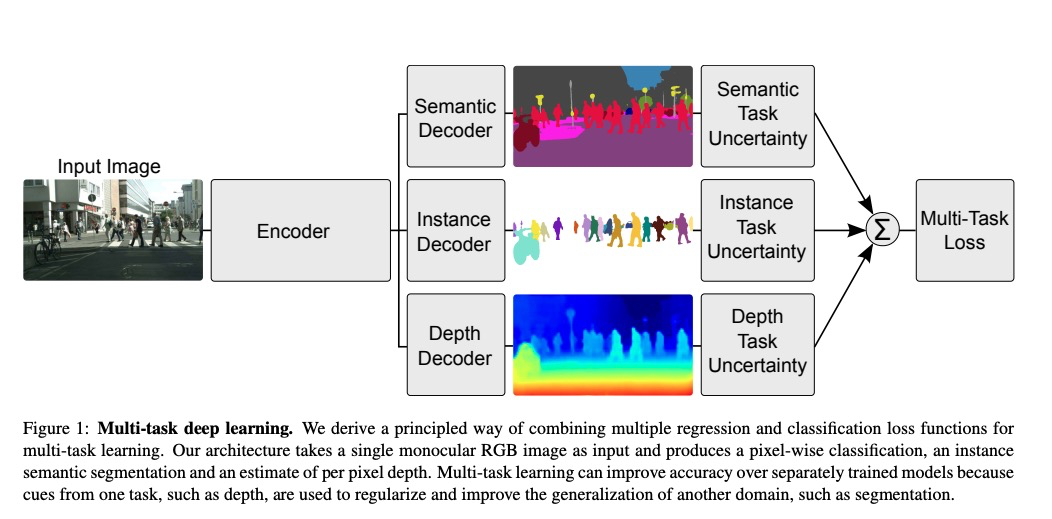总结来说，论文的关键贡献点：

1. 基于同方差不确定性，提出一个新颖且有指导性的多任务学习框架，可以同时学习不同尺度下的分类和回归任务；
2. 一个针对语义分割、实例分割和像素深度回归的统一框架；
3. 说明了在多任务学习中损失权重的重要性，且说明了对比单独训练模型，如何获得更优的模型性能。

## 2 相关工作

ignored

多任务学习涉及到一个关于多目标函数的模型的优化问题，这在深度学习中很普遍。融合多个目标函数的损失的简单方法是针对每个任务简单使用线性加权求和：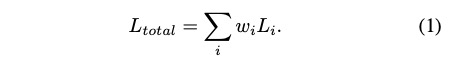这种方法在之前的工作中是占主流的。例如密集预测任务、场景理解任务、旋转任务（四元数）以及姿态平移任务等。这个方法存在一些问题，也就是，模型的性能对权重的选择非常敏感，如图2。这些权重超参数非常难以调节，通常每次任务需要花费数天时间。因此，非常迫切找到一种可以学习最优权重的简便方法。

更具体地说，我们来设计一个网络结构，它可以从一张图片中预测像素深度和语义类别。图2列出了每张图的边界表示每个任务的训练情况，曲线表示每个任务权重变化时的模型性能。可以看出，在几个较优权重处，联合任务的性能要比各个任务单独训练时的效果好。在最优权重附近，这个网络的性能劣于比其中一个任务的性能。然而，搜素这种最优权重成本高昂，且随着多任务大模型，难度递增。图2也累出了两种回归任务的相似结果，实例分割和像素深度回归。接下来，我们给出如何从概率模型中学习最优任务权重。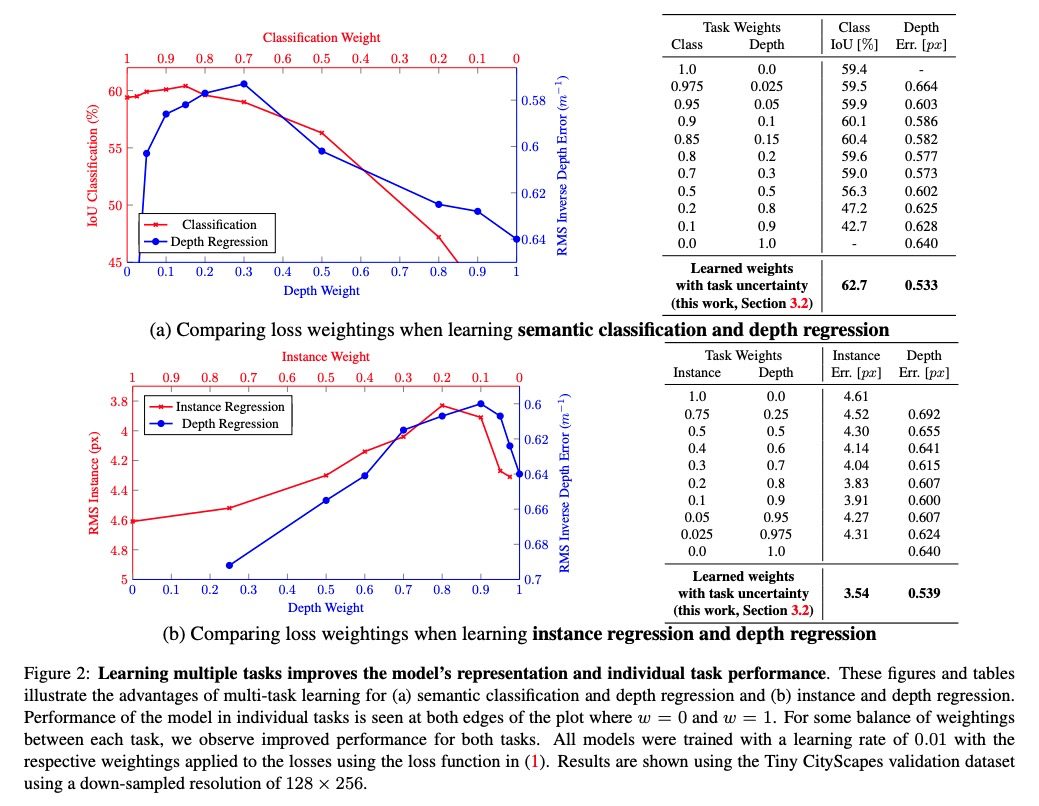### 3.1 同方差不确定性作为任务依赖不确定性

贝叶斯建模中存在的两类不确定性。

认知不确定性。缺乏训练数据导致的，可以通过增加数据来解释。

偶然不确定性。由于训练数据无法解释信息而导致的不确定性。偶然不确定性可以通过观测高准确率下的所有可解释变量来说明。偶然不确定性又可以分为两类：

数据依赖或异方差不确定性：依赖于输入数据，可以作为模型输出被预测出来。

任务依赖或同方差不确定性：不依赖于输入数据，不是模型输出，而是一个变量，对于输入数据保持不变，随着任务的不同而变化。

多任务场景中，我们发现任务不确定性可以捕获任务之间的相对置信度，反映了分类或回归任务的内在不确定性。它还依赖与任务的表征或度量单位。我们提出在多任务学习中可以使用同方差不确定性作为一个基准，来加权不同任务的损失。

### 3.2 多任务似然

基于最大化同方差不确定性的高斯似然估计，产生多任务损失函数。表示在权重为，输入为条件下的神经网络输出，对于回归任务，高斯似然估计为（为观测到的噪声标量）：

对于分类任务，通常会经过一个softmax变换，高斯似然估计为：

在多任务的这个例子中，我们将最大似然分解成独立因子，定义为充分统计量，可以得到多任务最大似然

为模型的输出（比如，语义分割，深度回归等）。

在最大似然推断中，我们最大化对数似然。回归模型中，对数似然可以表示为：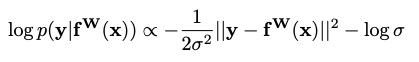对于高斯似然（或拉普拉斯相似度） ，然后我们可以在下最大化对数似然。

假定我们的模型输出有组成，服从如下高斯分布：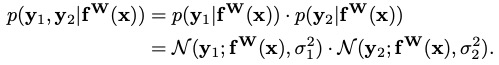损失函数

这里，为第一个输出变量的损失，同样如此。

极小化目标函数，可以把分别看做的相对权重，可根据数据自适应调整，变量的噪声参数增加的话，的权重降低。相应地，噪声降低的话，对应的损失函数权重增加。最后一项作为正则项，控制噪声增加过多（实际上或略了数据）。

这种设计可以拓展到更多的回归任务。对于分类任务，缩放后接softmax函数。如下：

这可以解释为玻尔兹曼分布（也叫Gibbs分布），输入除以（可以联系到温度T），这个尺度因子可固定或学习，参数的量级决定了这个离散分布的一致程度。这和用熵度量的不确定性相关。

对数似然可以表示为：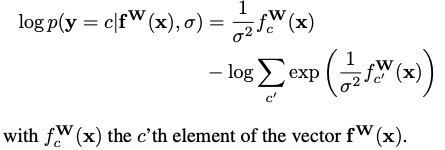接下来，假定多任务有连续输出任务 和离散输出任务构成，分别用高斯似然和对数似然表示。联合损失可以表示为：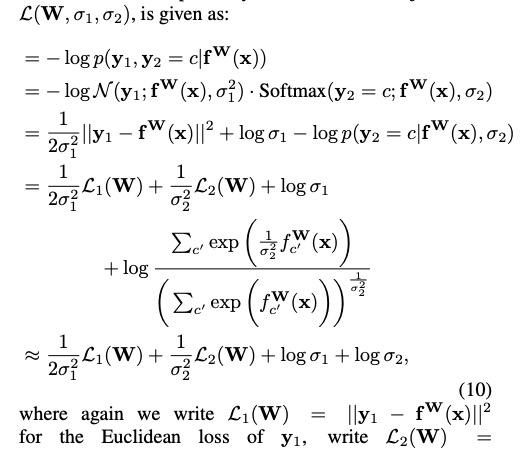为可学习的参数（在训练过程中，由于相较于更加稳定，并且由于作为损失函数权重系数的除数，采用尽可能的避免了除数为0的情况，因此，回归权重系数的目标变为了代替）。

## 4 视觉场景理解模型

我们设计了一个学习回归和分类任务的网络结构，包括几个深度卷积encoder层，后接对应任务的decoder卷积层。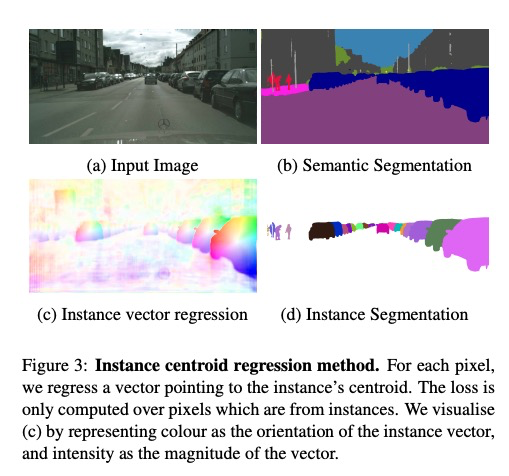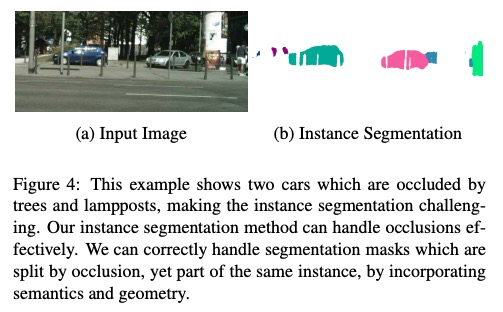## 5 实验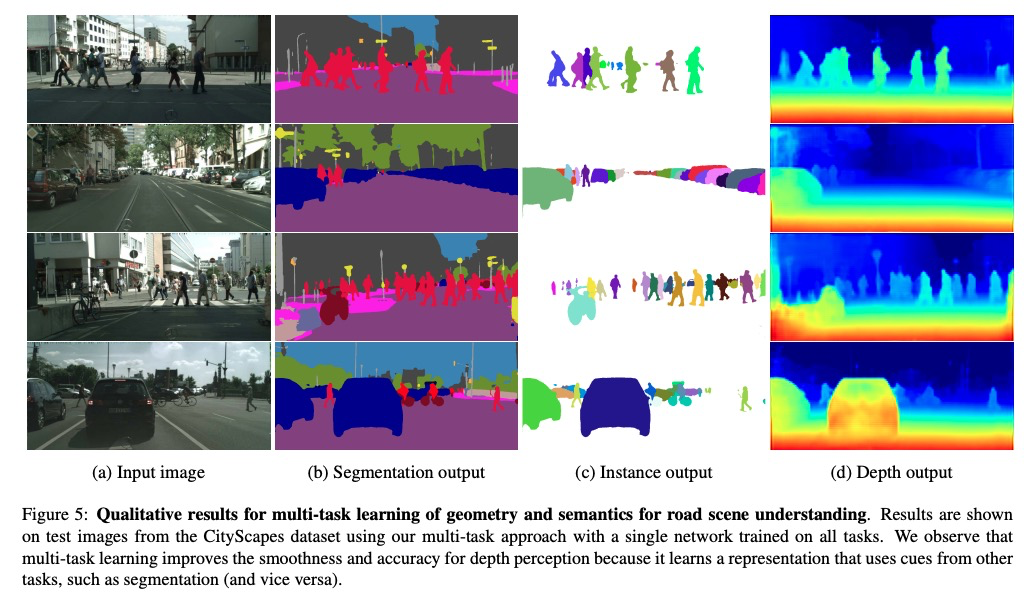## 6 结论

同方差不确定性是一种对损失加权的高效方法。任务依赖性的同方差不确定性可以提高模型的表达能力和各自任务的性能。

仍然有一些待遗留问题，

首先，对所有任务，最优权重不止一个。

第二个问题，将shared encoder网络，针对每个任务，拆分成单独的decoder的最佳位置在哪里？

最后一个问题，语义分割和深度预测的效果为何比语义分割是实例分割的效果更好？

展开全文自然语言处理 人工智能 深度学习 神经网络
• trafficlightdetectionUsingpythonopencv_红绿灯识别_yolov3_yolov3.weigh
• 论文题目：Multi-Task Learning Using Uncertainty to Weigh Losses for Scene Geometry and Semantics 中文翻译：使用不确定性来衡量权重损失的多任务学习——用于几何和语义场景 ...
论文题目：Multi-Task Learning Using Uncertainty to Weigh Losses
for Scene Geometry and Semantics
中文翻译：使用不确定性来衡量权重损失的多任务学习——用于几何和语义场景


# 摘要

只专注于单个模型可能会忽略一些相关任务中可能提升目标任务的潜在信息，通过进行一定程度的共享不同任务之间的参数，可能会使原任务泛化更好，许多深度学习应用受益于多任务学习——多元回归和目标分类。在本篇论文中，我们观察到不同任务的损失之间的尺度差异非常大，我们不能直接将各个任务的损失相加作为整体的损失，这样会导致一个任务收敛的很快，其他的任务表现很差，一个简单的解决方案是，将损失简单相加替换为加权和，以使所有任务损失的尺度接近。但是，这引入了需要进行调节的超参数。而对这些加权值进行手工微调是一件很困难且昂贵的事情，会使得多任务学习在实践中让人望而却步。由此我们提出了一种新的确定每个任务加权值的方法——引入同方差的不确定性来确定 MTL 中损失的权重：在每个任务的损失函数中学习另一个噪声参数（noise parameter）。此方法可以接受多任务（可以是回归和分类），并统一所有损失的尺度。这样，我们就能像一开始那样，直接相加得到总损失了。

# 1. Introduction

多任务学习通过从共享的特征表达中学习多个目标来提高学习效率和预测精度，多任务学习现在已经应用在机器学习的方方面面——从计算机视觉到自然语言处理到语言识别。

我们在计算机视觉的环境理解中探索多任务学习，环境理解算法必须同时理解环境的几何和语义信息，这引入了一个有趣的多任务问题——环境理解涉及到不同单元和尺度的各种分类和回归任务的联合学习，视觉环境理解的多任务学习在系统中是非常重要的，因为在这样的系统中长时间的计算力投入是禁止的，比如机器人中。将所有的任务结合成一个模型可以减少计算力，并且允许这些系统实时运行。

之前同时学习多个任务的方法是简单使用损失的加权和，损失的加权值或是均匀的，或是需要手工微调，然而，我们证明了这样做的模型的表现强烈依赖于每个任务损失的加权值，而手工去微调这些加权值达到最优是很昂贵且困难的。我们观察到每个任务最佳权重依赖于一个衡量尺度——最后发现这个尺度就是任务噪声的幅度。

在这篇工作中，我们提出了一个原则性的方法——使用同方差不确定性来将多个任务损失结合来同时学习多个目标，我们将同方差不确定性看作和任务相关的加权值，同时展示了怎样得到一个原则性的多任务损失函数，它可以很好的平衡不同回归和分类损失，我们的方法可以学着去平衡这些加权值，与单独学习每个任务相比，产生了更加优越的性能。

具体来讲，我们展示了在学习环境几何和语义等三个任务中我们方法的表现。首先，我们学着去针对每个像素点对目标分类（称之为语义分割），其次，我们的模型执行实例分割，这是更难的任务——因为它不仅需要判断每个像素的类别，还需要判断这个像素点属于哪个目标。这比单独的目标检测也要难。最后，我们证明了使用语义标签和多任务深度学习可以提高几何和深度估计。

在现有文献中，经常使用单独的深度学习模型来学习深度回归、语义分割和实例分割来构建一个完整的系统。给一张单目的输入图片，我们的系统首先去做语义分割、实例分割和深度密集估计（如Figure 1所示）。而其他的视觉模型已经展示了多任务学习，我们展示了如何将几何和语义信息结合起来，将这些任务结合起来构成单个模型确保了在减少计算的同时完成单个任务的输出，最后，我们展示了多任务中的共享表达可以提高在不同指标上的性能，来使得模型更高效：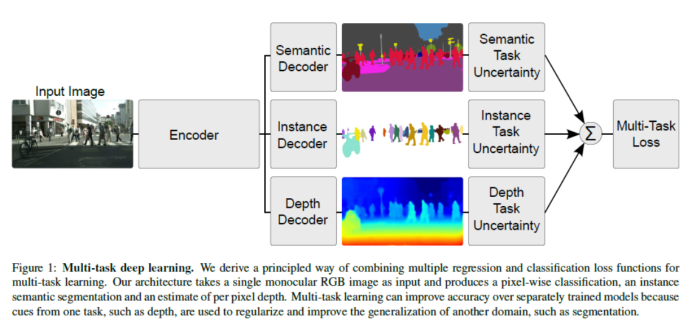总的来说，本篇论文的主要贡献在于：

1. 一个新颖且原则性的多任务损失使用同方差不确定性来同时学习不同数量和单元的各种分类和回归损失；
2. 一个用来完成语义分割、实例分割和 depth regression；
3. 展示了多任务学习中损失加权值得重要性，相比于单独训练每个模型，我们如何才能通过多任务学习获得更优越的表现；

# 2. Multi Task Learning with Homoscedastic Uncertainty

多任务学习涉及到优化到多个目标，是现在深度学习很流行的一个问题。原来将多个目标损失结合在一起的方法就是对单个任务的损失简单多一个加权和：这是之前的工作中使用的主要方法，其中模型的表现力对于 w i w_{i} 特别敏感（如上面的Figure 2所示），这些加权超参数手工调参代价很高，因此发现一种便利的方法来学习最佳加权值是很迫切的。

更具体的讲，我们考虑一个网络来学习从输入图片中预测像素深度和语义类别。在Figure 2中每张图的边界表示分别在单个任务上训练的模型，曲线刻画了对于不同权重 w i w_{i} 的表现，我们观察到在一些最佳加权值中，这种联合网络表现得比分别训练每个网络要好。Figure 2也展示了两个回归任务的相似结果：实例分割和深度回归。我们接下来展示了如何使用概率建模的想法来学习最佳任务权重。

## 3.1. 同方差不确定性（任务依赖型不确定性）

在贝叶斯建模中，有两种不同类型的不确定性可以建模。

• 认知不确定性是模型中常见的一种不确定性，通常由数据不足产生，可以通过增加训练数据来解释，认知不确定性测量的，是我们的input data是否存在于已经见过的数据的分布之中；
• 偶然不确定性捕捉到的是我们的数据不能解释的信息；

偶然不确定性可以再分为两个子类：

• 数据依赖型（不同方差）是一种依赖于输入数据的偶然不确定性，可以作为一个模型输出预测；
• 任务依赖性（相同方差）是不依赖于输入数据的偶然不确定性，它不是一个模型输入，对于所有输入数据来说都是常量，但是不同任务之间不同。因此被描述为任务依赖型不确定性；

在多任务设置中，我们展示了任务不确定性捕捉到了任务之间的相关置信度，反映了回归和分类任务的不确定性，它也依赖于任务的表征和衡量单元，我们提出了我们可以使用同方差不确定性作为多任务学习问题中加权损失的基础。

在本节中，我们提出了基于最大化同方差高斯似然函数的多任务损失函数，我们令 f W ( x ) f^{W}(x) 作为输入x权重W的神经网络输出，我们定义了下面的概率模型，对于回归任务，我们讲我们的似然函数定义为高斯分布（均值为模型输出，方差为噪声）：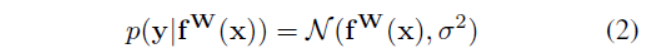加入了一个噪声因子 σ \sigma ，对于分类任务我们经常用softmax函数压缩模型输出，并从结果概率向量中采样：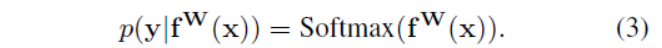在有多个模型输出的情况下，我们经常多任务似然函数定义为：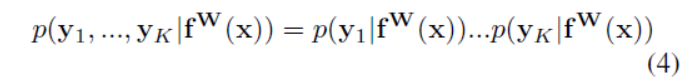模型输出为 y 1 , y 2 . . . y K y_{1},y_{2}...y_{K} f W ( x ) f^{W}(x) 为我们充足的统计，其实就是将网络看作一个作用在输入上的函数f。

在最大化似然函数中，我们一般选择最大化对数似然函数，在回归问题中，举个例子，对数似然函数可以写为：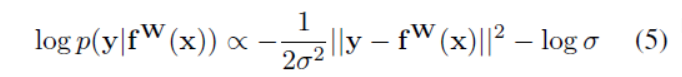对于高斯似然， σ \sigma 是模型观察到的噪声参数——捕捉到了输出中有多少噪声，接着我们最大化和模型参数W和观察噪声参数 σ \sigma 有关的对数似然函数。

让我们现在假设模型输出由两个向量 y 1 和 y 2 y_{1}和y_{2} 组成，每一个都遵循高斯分布：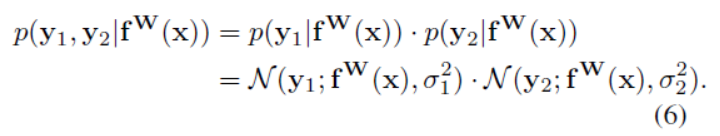最大化似然函数（6）相当于最小化对数似然函数的相反数，于是我们要最小化的多目标输出模型为 £ ( W , σ 1 , σ 2 ) \pounds (W,\sigma _{1},\sigma _{2})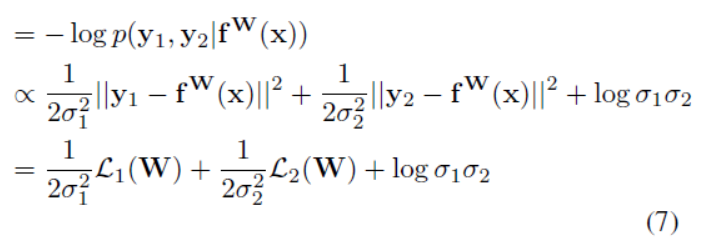这里我们将 £ 1 ( W ) = ∣ ∣ y 1 − f W ( x ) ∣ ∣ 2 \pounds _{1}(W)=||y_{1}-f^{W}(x)||^{2} 记为第一个输出变量的损失， £ 2 ( W ) \pounds _{2}(W) 类似。

我们通过最小化（7）式，来自适应地学习损失 £ 1 ( W ) 和 £ 2 ( W ) \pounds _{1}(W)和\pounds _{2}(W) 的相对权重 σ 1 和 σ 2 \sigma _{1}和\sigma _{2} 。当 变 量 y 1 变量y_{1} 的噪声参数 σ 1 \sigma _{1} 增加时意味着损失 £ 1 ( W ) \pounds _{1}(W) 的加权值降低了，另一方面，当噪声减少时，目标损失的相关权重增大了，同时通过（7）式中的最后一项来限制 σ 1 和 σ 2 \sigma _{1}和\sigma _{2} ，使得其不能太大，起了一个正则化的作用。这个地方我是这么想的，噪声参数大意味着输出变量的方差太大，结果不可靠，所以在目标损失中该损失的加权值应该减少。

这种结构可以简单扩展到多个回归输出中，然而，扩展到分类似然函数中更有趣，我们将噪声参数 σ \sigma 作为一个缩放因子加入到（3）式中：σ \sigma 是一个正因子，这个可以看成是一个玻尔兹曼分布（输入被放缩了 σ 2 倍 \sigma ^{2}倍 ），这个因子可以是固定的，也可以学习得到，参数的幅度决定了离散分布的均匀性，这和不确定性有关，输出的对数似然可以被写为：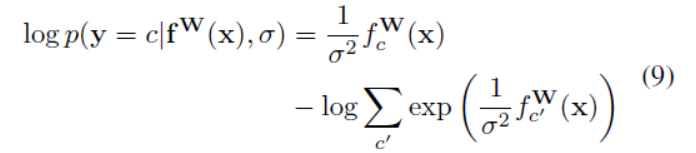f c W f^{W}_{c} 可以看作是向量 f W f^{W} 的第c个元素。

接下来，我们假设模型的多个输出由一个连续的输出 y 1 y_{1} （回归值）和离散的输出 y 2 y_{2} （分类值）组成，分别由高斯似然和softmax似然建模，就像之前定义一个联合损失 £ ( W , σ 1 , σ 2 ) \pounds(W,\sigma _{1},\sigma _{2}) ，可以写成：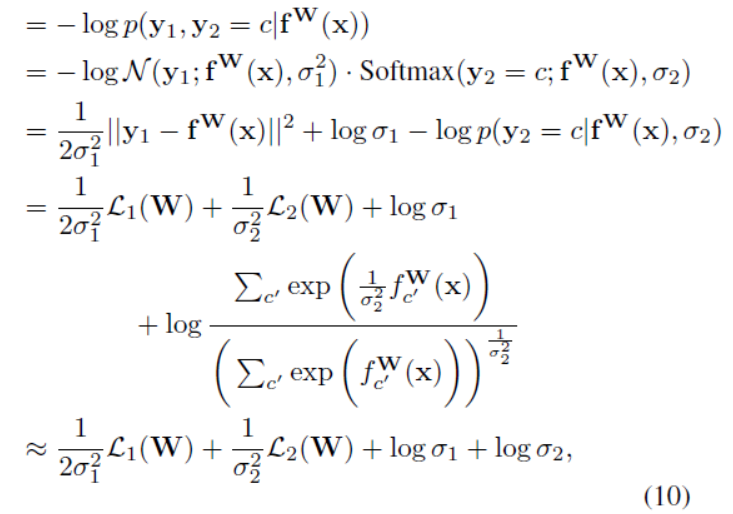这里的 £ 1 ( W ) = ∣ ∣ y 1 − f W ( x ) ∣ ∣ 2 ， £ 2 ( W ) = − l o g S o f t m a x ( y 2 , f W ( x ) ) \pounds _{1}(W)=||y_{1}-f^{W}(x)||^{2}，\pounds _{2}(W)=-logSoftmax(y_{2},f^{W}(x)) ，这里观察到当 σ 2 \sigma _{2} 趋向于1时，最后一项可以通过 1 σ 2 ∑ c ′ e x p ( 1 σ 2 2 f c ′ W ( x ) ) ≈ e x p ( f c ′ W ( x ) ) 1 σ 2 2 \frac{1}{\sigma _{2}}\sum _{c^{&#x27;}}exp(\frac{1}{\sigma ^{2}_{2}}f^{W}_{c^{&#x27;}}(x))\approx exp(f^{W}_{c^{&#x27;}}(x))^{\frac{1}{\sigma ^{2}_{2}}} 来近似，这极大地简化了优化目标。

这种结构可以到连续和离散损失函数的任意结合，允许我们以一种原则性的方法学习每个损失的相关权重，这种损失可以平滑地区分，相关权重不会收敛到0。相反，使用（1）中简单地加权和会导致相关权重可能收敛到0。

在实践中，我们训练网络来预测对数方差 s : = l o g σ 2 s:=log\sigma ^{2} ，这是因为回归s比回归 σ 2 \sigma ^{2} 更稳定，这样也可以避免分母项为0，同时指数映射也允许来回归没有约束的缩放值，这里的exp(-s)被解析到正值，给了方差一个有效值。

展开全文多任务学习
•    Multi-Task Learning Using Uncertainty to Weigh Losses for Scene Geometry and Semantics   个人理解：我们使用传统的多任务时，损失函数一般都是各个任务的损失相加，最多会为每个任务的损失前添加...
• Multi-Task Learning Using Uncertainty to Weigh Losses for Scene Geometry and Semantics https://arxiv.org/abs/1705.07115 CVPR 2018 Alex Kendall University of Cambridge Yarin Gal University of Oxford.....深度学习 multi task
• 称 重 设计 参考（选型、ADC精度、噪声、漂移）等因素 a-reference-design-for-weigh-scales
• 前端开源库-weighweight，一个命令行工具，用于检查任何浏览器兼容的NPM模块的包大小。
• very useful forex indicator
• 特拉维斯称重跟踪每个提交/拉取请求如何影响回购中文件的大小。 您就是您要测量的东西！ 当每个Pull Request更改了生成的文件（例如，缩小JavaScript包）并在GitHub存储库中提交时，该脚本可让您跟踪生成的文件的...Python
•Go
• 本文提出了一种多任务loss权重学习的方式，通过训练协调了三个任务的loss，有语义分割、实力分割和depth regression，结构主要是： 文中主要分为了三种情况来介绍： 回归任务 定义一个概率分布函数，假设其符合...pytorch 神经网络 深度学习
• 微纳尺度下Al/Cu复合界面热导率和原子量之间关系的数值研究，葛道晗，，本文基于非平衡分子动力学法系统研究了Al/Cu界面的界面热导率，并考虑了原子迁移及不同温度下原子重力的影响。研究发现材料的界面�
• 算法效率衡量 执行时间反应算法效率 对于同一问题，我们给出了两种解决算法，在两种算法的实现中，我们对程序执行的时间进行了测算，发现两段程序执行的时间相差悬殊（214.583347秒相比于0.182897秒），由此我们...算法
• The balance was the first mass measuring instrument invented. In its traditional form, it consists of a pivoted horizontal lever of equal length arms, called the beam, with a weighing pan, also called...
• 多任务学习系统的性能很大程度上依赖于任务间的损失权值。手动调节权值非常耗时耗力，论文提出使用任务间的同方差不确定性给每个损失函数赋权。模型由单一图像同时学习像素级深度回归、语义及实例分割。...
•多任务学习...